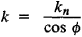# Normal Section

## normal section

[′nȯr·məl ′sek·shən]
(mathematics)
Relative to a surface, this is a planar section produced by a plane containing the normal to a point.
McGraw-Hill Dictionary of Scientific & Technical Terms, 6E, Copyright © 2003 by The McGraw-Hill Companies, Inc.
The following article is from The Great Soviet Encyclopedia (1979). It might be outdated or ideologically biased.

## Normal Section

A normal section of a surface S at a given point M on the surface is the curve of intersection of S with a plane drawn through the normal at the point M. Normal sections are used to study the curvature of S in different (tangential) directions at M. Among these there are two (mutually perpendicular) directions, called principal, for which the normal curvature, that is, the curvature of the corresponding normal section, attains its maximum and minimum values k1 and k2 (the principal curvatures at the given point). The curvature of a normal section is assigned a plus (minus) sign if the direction of concavity of the section coincides with (is opposite to) the positive direction of the normal to the surface. The normal curvature of a surface in a given direction can be expressed very simply in terms of the principal curvatures; specifically, the curvature kn of the normal section in a direction forming an angle ϕ with the first principal direction is related to k1 and k2 by the expression

kn = k1 cos2 ϕ + k2 sin2 ϕ

which is Euler’s theorem on normal curvature. The curvatures of oblique sections of a surface are also studied by using the curvatures of normal sections. Specifically, the curvature k of an oblique section of a plane a that passes through a given tangent line a is expressed by Meusnier’s formulawhere ϕ is the angle between the plane α and the normal to the surface and kn is the normal curvature of the surface in the direction of line a.

References in periodicals archive ?
The normal section and college day will now be compressed into a half day, following the end of the AGM.
Limited Crack Width in Normal Section. According to the limit stipulated in the Chinese Code GB/T 50152 (2012) , specimen would be considered as damaged when the width of normal section crack reaches 1.5 mm.
"By registering case under normal section the case was being diluted", he said
As we know from mechanics of solid deformable body for the task we need two main and four additional conditions, there are two equilibrium equations for planar systems in a normal section, as well as auxiliary terms in the form of strain distribution law along the section height of the element 1/r = ([[epsilon].sub.c] + [[epsilon].sub.s])/d, physical dependence between stresses in the reinforcement [[sigma].sub.s] = f([[epsilon].sub.c]), and the compressed and tensioned zones of the concrete [[sigma].sub.c] = f([[epsilon].sub.c]) and [[sigma].sub.ct] = f([[epsilon].sub.ct]).
Normal section fracture of flexed reinforced concrete structures starts with the reinforcement reaches the yielding stress.
For a normal Section 232/223(f) purchase or refinance loan, the escrow for repairs and improvements may constitute up to 15 percent of the loan amount, so many owners view these loans as a good option for properties that need certain repairs and replacements to maintain condition and competitiveness.
The project envisages construction of 13.9 KM long dedicated signal free corridor of 9.60 to 10.10 meters width at normal section and from 19.00 to 21.10 meters width at Metro Bus System (MBS) stations and trenches in Islamabad Capital Territory.
It is curious to note that Police administration took case under the normal sections of penal code which we have previous knowledge regarding ultimate result of the those cases, sometimes those perpetrators get bail on the plea of normal section of law resulting impunity.
If, for a normal section V on M, we have [A.sub.V] = [alpha]I, where a is a differentiable function and I is the identity morphism on [GAMMA](TM), then M is called totally umbilical with respect to any normal section V.
The cylindrical worm having the flanks as helical surfaces of a convolute helicoid of the second specie has the property that in the normal section on the reference medium helix of the tooth or of the tooth space.
The fiber direction in the injection axis reference (1,2,3) is given by the two angles [empty set]' and [theta]', which can be determined by the measurement of ellipse dimensions d, a, and [empty set] in the normal section plane (see Fig.

Site: Follow: Share:
Open / Close